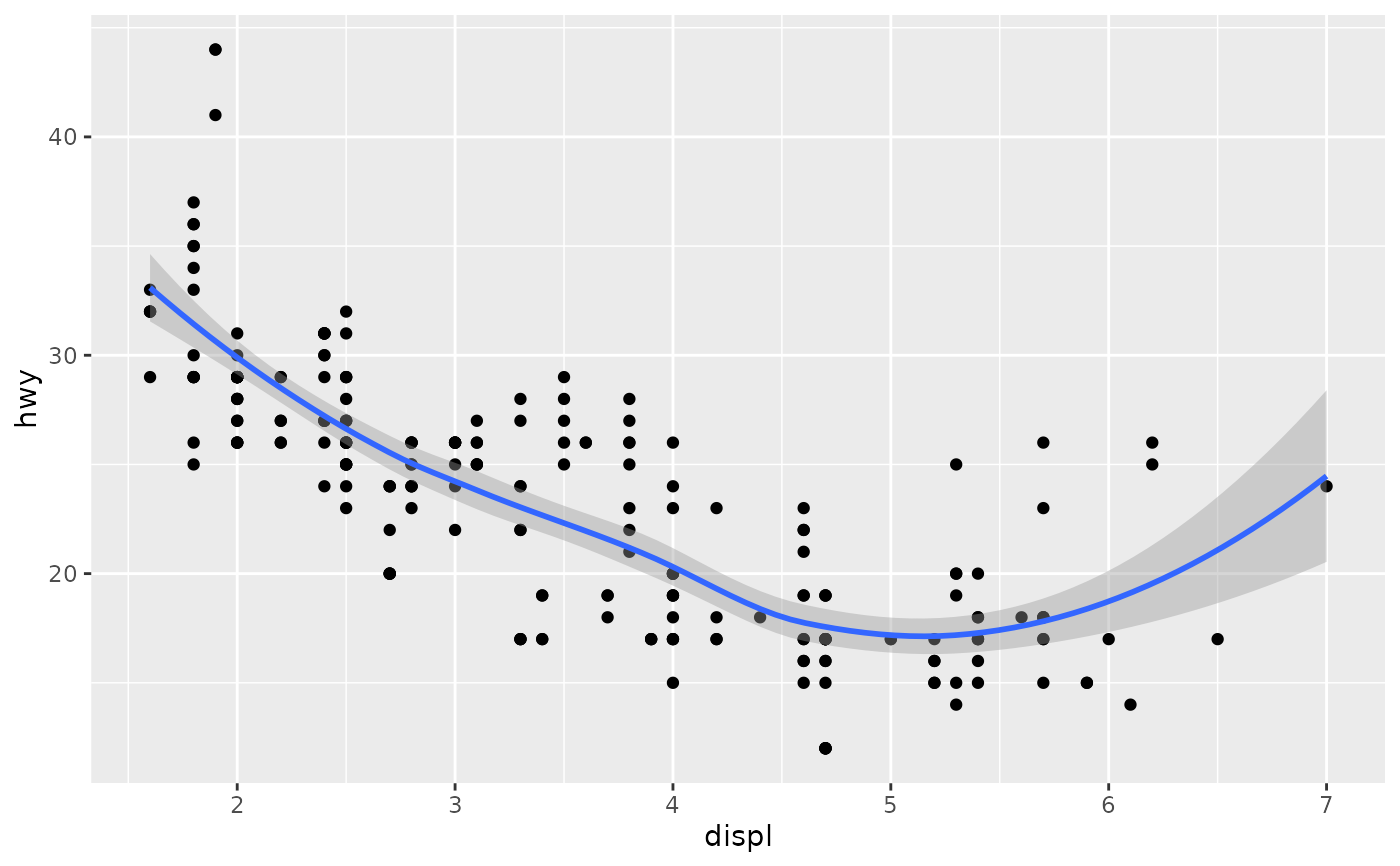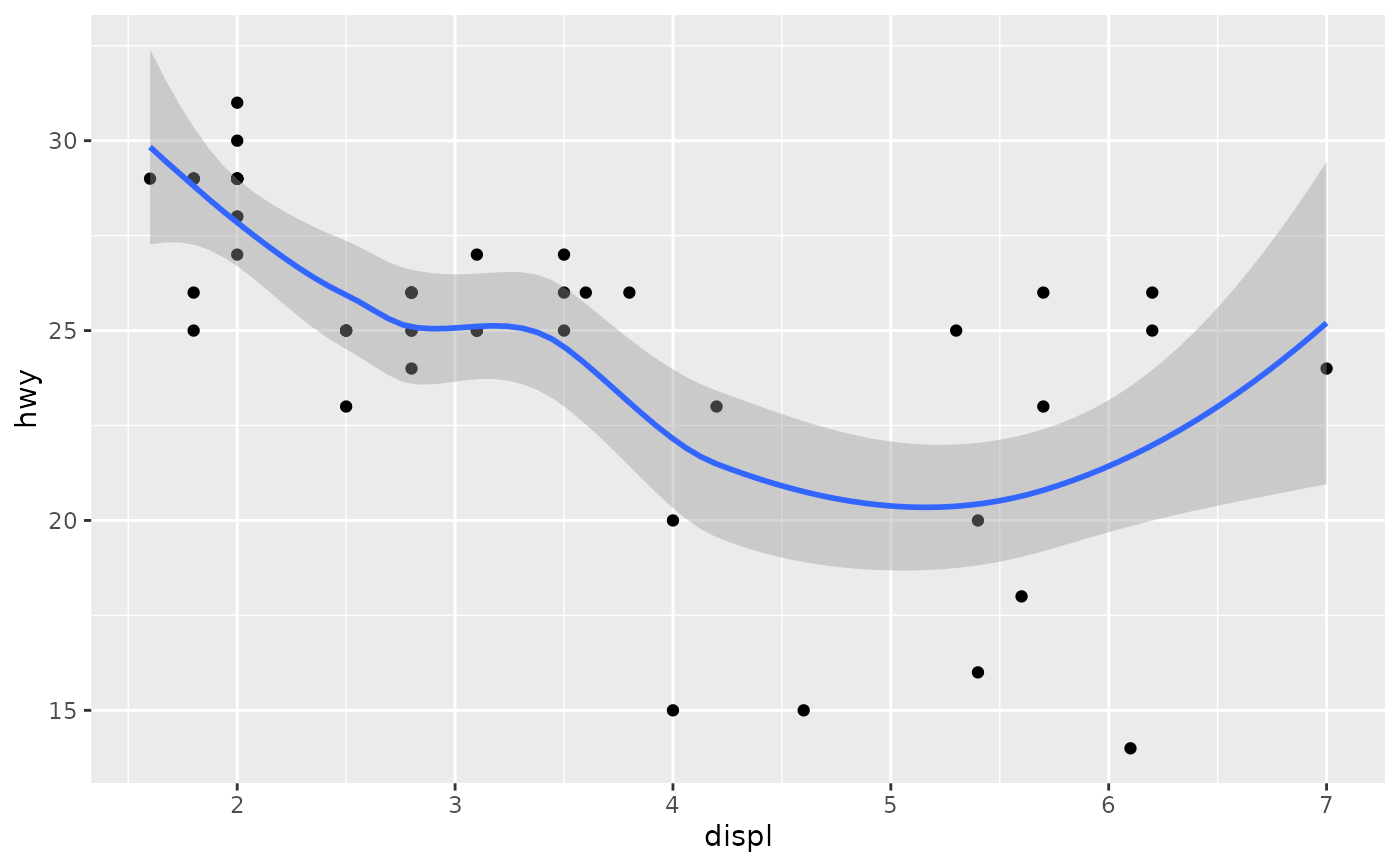+ is the key to constructing sophisticated ggplot2 graphics. It allows you to start simple, then get more and more complex, checking your work at each step.

## Usage

# S3 method for gg
+(e1, e2)

e1 %+% e2

## Arguments

e1

An object of class ggplot() or a theme().

e2

A plot component, as described below.

You can add any of the following types of objects:

• An aes() object replaces the default aesthetics.

• A layer created by a geom_ or stat_ function adds a new layer.

• A scale overrides the existing scale.

• A theme() modifies the current theme.

• A coord overrides the current coordinate system.

• A facet specification overrides the current faceting.

To replace the current default data frame, you must use %+%, due to S3 method precedence issues.

You can also supply a list, in which case each element of the list will be added in turn.

theme()

## Examples

base <-
ggplot(mpg, aes(displ, hwy)) +
geom_point()
base + geom_smooth()
#> geom_smooth() using method = 'loess' and formula = 'y ~ x'# To override the data, you must use %+%
base %+% subset(mpg, fl == "p")# Alternatively, you can add multiple components with a list.
# This can be useful to return from a function.
base + list(subset(mpg, fl == "p"), geom_smooth())
#> geom_smooth() using method = 'loess' and formula = 'y ~ x'